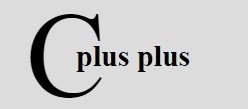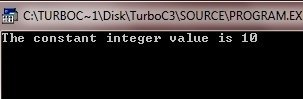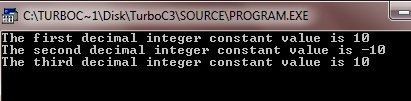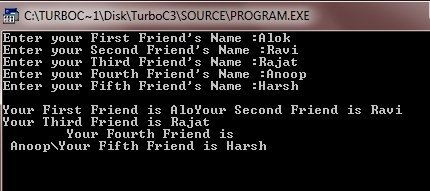# C++ Constants

Constants are the data items that never change their value during a program run.

## C++ Constants Example

Following is an example program of C++ constants :

```/* C++ Constants or Literals Example */

#include<iostream.h>
#include<conio.h>

void main()
{
clrscr();

const int num=10;

cout<<"The constant integer value is "<<num;

getch();
}```

When the above C++ Program is compile and executed, it will produce the following output:C++ allows the following kinds of literals :

• integer-constant
• character-constant
• floating-constant
• string-literal

## C++ Integer Constants

Integer constants are whole numbers without any fractional part.

Method to write integer constant is - An integer constant must have at least one digit and must not contain any decimal point. It may contain either + or - sign. A number with no sign is assumed to be positive. Commas cannot appear in an integer constant.

C++ allows the following three types of integer constants :

• Decimal ( base 10 )
• Octal ( base 8 )
• Hexadecimal ( base 16 )

Let's discuss all the three types one by one.

### C++ Decimal Integer Constants

An integer constant consisting of a sequence of digits is taken to be decimal integer constant unless it begins with 0 ( digit zero ). For instance, 1234, 43, +85, -25 are decimal integer constants

#### C++ Decimal Integer Constants Example

Following is the example program of C++ decimal integer constants :

```/* C++ Decimal Integer Constant Example */

#include<iostream.h>
#include<conio.h>

void main()
{
clrscr();

const int nump=+10, numm=-10, numn=10;

cout<<"The first decimal integer constant value is "<<nump;
cout<<"\nThe second decimal integer constant value is "<<numm;
cout<<"\nThe third decimal integer constant value is "<<numn;

getch();
}```

When the above C++ program is compile and executed, it will produce the following output:### C++ Octal Integer Constants

A sequence of digits starting with 0 ( digit zero ) is taken to be an octal integer. For instance, decimal integer 8 will be written as 010 as octal integer. (... 810 = 108) and decimal integer 12 will be written as 014 as octal integer (...1210 = 148)

The example program for C++ octal integer constants is same as above, but the number in this case is in octal form.

To convert a number from any number system to octal number system and vice-versa check the following:

### C++ Hexadecimal Integer Constants

A sequence of digits preceded by 0x or 0X is taken to be an hexadecimal integer. For instance, decimal 12 will be written as 0XC as hexadecimal integer.

Thus number 12 will be written either as 12 ( as decimal ), 14 ( as octal ) and 0XC ( as hexadecimal ).

The suffix l or L and u or U attached to any constant forces it to be represented as a long and unsigned respectively.

The example program for C++ hexadecimal integer constants is same as above, but the number in this case is in hexadecimal form.

To convert a number from any number system to hexadecimal number system and vice-versa check the following:

## C++ Character Constants

A character constant is one character enclosed in single quotes, as in 'z'.

The rule to write character constant is - A character constant in C++ must contain one character and must be enclosed in single quotation mark.

### C++ Escape Sequences

C++ allows some non-graphic characters in character constant. Nongraphic characters are those characters that cannot be typed directly from keyboard. For example, backspace, tabs, carriage return etc. These nongraphic characters can be represented by using escape sequences. An escape sequence is represented by a backslash (\) followed by one or more characters. Following table lists the C++ Escape Sequences :

Escape Sequence Nongraphic Character
\a Audible bell (alert)
\b Backspace
\f Formfeed
\n Newline or linefeed
\r Carriage Return
\t Horizontal tab
\v Vertical tab
\\ Backslash
\' Single quote
\" Double quote
\? Question mark
\On Octal number (On represents the number in octal)
\xHn Hexadecimal number (Hn represents the number in hexadecimal)
\0 Null

Note : An escape sequence represents a single character and hence consumes one byte in ASCII representation.

#### C++ Escape Sequences Example

Following is the example program of C++ escape sequence :

```/* C++ Escape Sequences Example */

#include<iostream.h>
#include<conio.h>

void main()
{
clrscr();

char name1, name2, name3;
char name4, name5;

cout<<"Enter your First Friend's Name :";
cin>>name1;

cout<<"Enter your Second Friend's Name :";
cin>>name2;

cout<<"Enter your Third Friend's Name :";
cin>>name3;

cout<<"Enter your Fourth Friend's Name :";
cin>>name4;

cout<<"Enter your Fifth Friend's Name :";
cin>>name5;

cout<<"\nYour First Friend is "<<name1;
cout<<"\bYour Second Friend is "<<name2;
cout<<"\nYour Third Friend is "<<name3;
cout<<"\n\tYour Fourth Friend is\n "<<name4;
cout<<"\\Your Fifth Friend is "<<name5;

getch();
}```

When the above program is compile and executed, it will produce the following output:## Floating Constants

Floating constants are also called as real constants.

Real constants are numbers having fractional parts. These may be written in one of the two forms called fractional form or the exponential form.

A real constant in fraction form consists of signed or unsigned digits including a decimal point between digits.

The rule for writing a real constant in fractional form is - A real constant in fractional form must have at least one digit before a decimal point and at least one digit after the decimal point. It may also have either + or - sign preceding it. A real constant with no sign is assumed to be positive.

Following are some valid real constants in fractional form :

`1.0,   12.7,   -534.0,    -0.00234`

Following are some invalid real constants :

```8    	     (No decimal point)
8.   	     (no digit after decimal point)
+18/2  	     (/-illegal symbol)
18,250.23.5  (two decimal points)
18,230.262   (comma not allowed)```

A real constant in exponent form consists of two parts : mantissa and exponent. For instance, 5.8 can be written as 0.58 * 101 = 0.58 E01 where mantissa part is 0.58 (the part appearing before E) and exponent part is 1 (the part appearing after E). E01 represents 101.

The rule for writing a real constant in exponent form is - A real constant is exponent form has two parts : a mantissa and an exponent. The mantissa must be either an integer or a proper real constant. The mantissa is followed by a letter E or e and the exponent. The exponent must be an integer.

Following are some valid real constants in exponent form :

`152E05,  1.52E07,  0.152E08,  152.0E08,  152E+8,  1520E04,  -0.172E-3`

Following are some invalid real constants in exponent form:

```172.E5         (At least a digit must follow the decimal point)
1.7E           (No digit specified for exponent)
0.17E2.3       (Exponent can not have fractional part)
17.225E02      (No comma allowed)
.25E-7         (No preceding digits before decimal point)```

## String Constants (Literals)

'Multiple Character' constants are treated as string-literals.

The rule to write string-literal is - A string literal is a sequence of characters surrounded by double quotes.

The difference between multicharacter character constants (which are generally used for writing octal numbers or hexadecimal numbers or ASCII numbers) and string-literals is that multicharacer character constants are treated as integers (int type) and their values are implementation (version) dependent. Whereas a string-literal is treated as array of char. The benefit of array is that each constituent character of the string can be dealt with separately.

Each string literal is by default (automatically) added with a special character '\0' which makes the end of a string. '\0' is a single character. Thus the size of a string is the number of characters plus one for this terminator. For example,
"abc" size is 4.
"\ab" size is 3 (\a is an escape sequence, thus one character).
"Subhu\'s pen" size is 12 (For typing apostrophe(') sign escape sequence has been used).

Thus "abc" will actually be represented as "abc\0" in the memory i.e., along with the terminator character.

Tools
Calculator

Quick Links
Signup - Login - Give Online Test